Скачать презентацию Boolean Algebra Logic Circuit Boolean Algebra

d35d4319a4591a876a18ff8184e6f3d4.ppt

• Количество слайдов: 22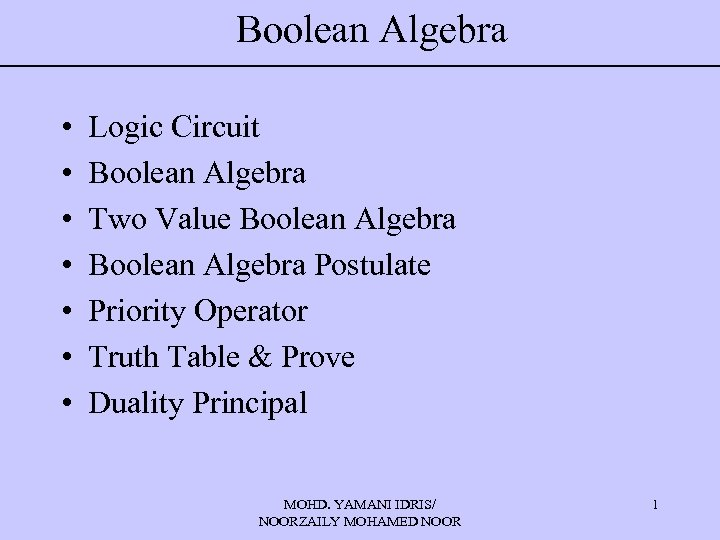Boolean Algebra • • Logic Circuit Boolean Algebra Two Value Boolean Algebra Postulate Priority Operator Truth Table & Prove Duality Principal MOHD. YAMANI IDRIS/ NOORZAILY MOHAMED NOOR 1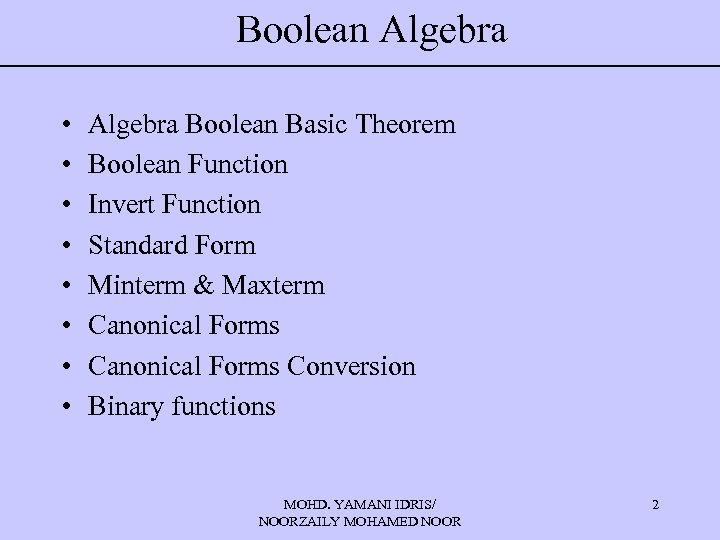Boolean Algebra • • Algebra Boolean Basic Theorem Boolean Function Invert Function Standard Form Minterm & Maxterm Canonical Forms Conversion Binary functions MOHD. YAMANI IDRIS/ NOORZAILY MOHAMED NOOR 2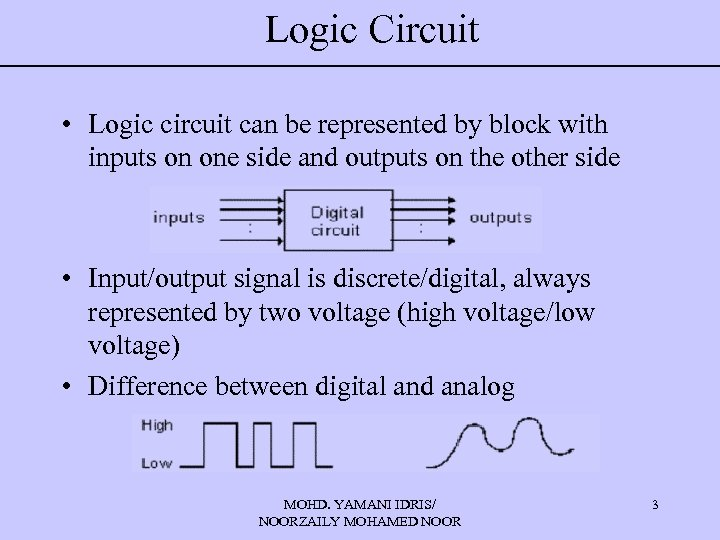Logic Circuit • Logic circuit can be represented by block with inputs on one side and outputs on the other side • Input/output signal is discrete/digital, always represented by two voltage (high voltage/low voltage) • Difference between digital and analog MOHD. YAMANI IDRIS/ NOORZAILY MOHAMED NOOR 3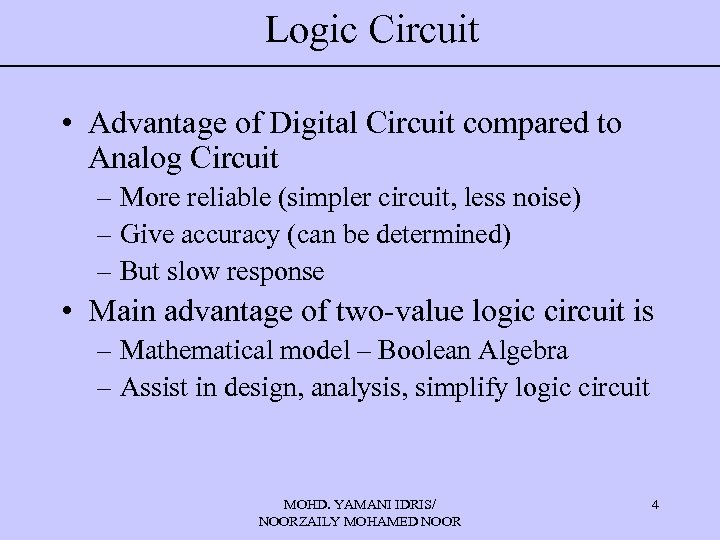Logic Circuit • Advantage of Digital Circuit compared to Analog Circuit – More reliable (simpler circuit, less noise) – Give accuracy (can be determined) – But slow response • Main advantage of two-value logic circuit is – Mathematical model – Boolean Algebra – Assist in design, analysis, simplify logic circuit MOHD. YAMANI IDRIS/ NOORZAILY MOHAMED NOOR 4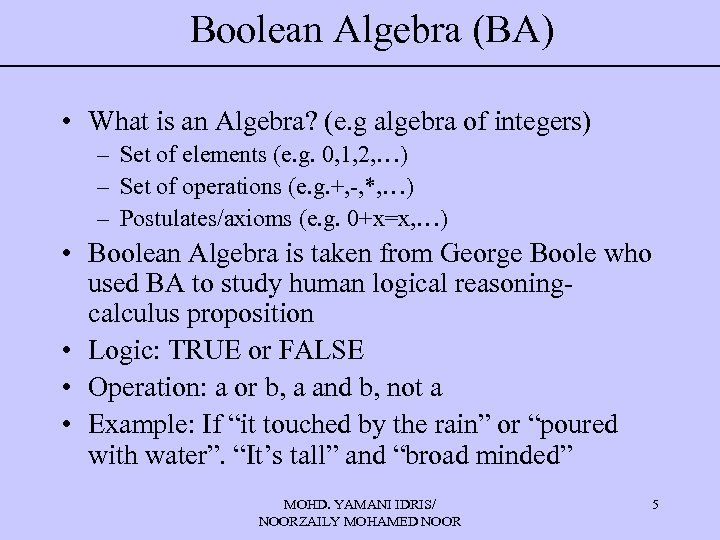Boolean Algebra (BA) • What is an Algebra? (e. g algebra of integers) – Set of elements (e. g. 0, 1, 2, …) – Set of operations (e. g. +, -, *, …) – Postulates/axioms (e. g. 0+x=x, …) • Boolean Algebra is taken from George Boole who used BA to study human logical reasoningcalculus proposition • Logic: TRUE or FALSE • Operation: a or b, a and b, not a • Example: If “it touched by the rain” or “poured with water”. “It’s tall” and “broad minded” MOHD. YAMANI IDRIS/ NOORZAILY MOHAMED NOOR 5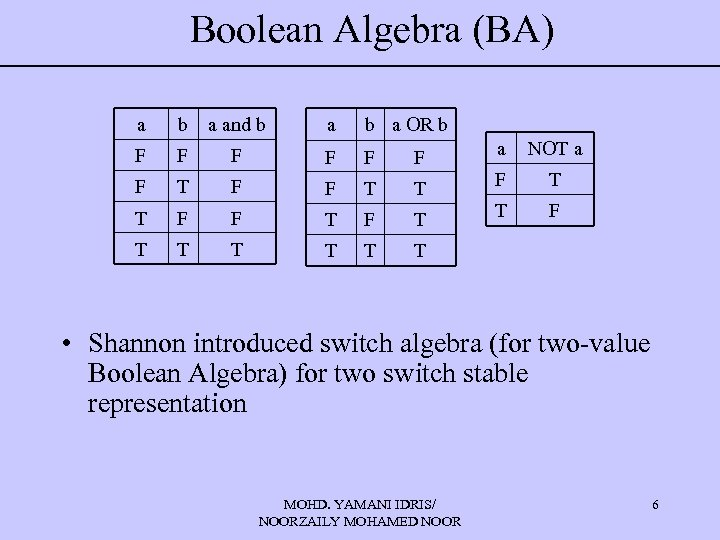Boolean Algebra (BA) a b a and b a OR b F F F a NOT a F T F F T T F T T T • Shannon introduced switch algebra (for two-value Boolean Algebra) for two switch stable representation MOHD. YAMANI IDRIS/ NOORZAILY MOHAMED NOOR 6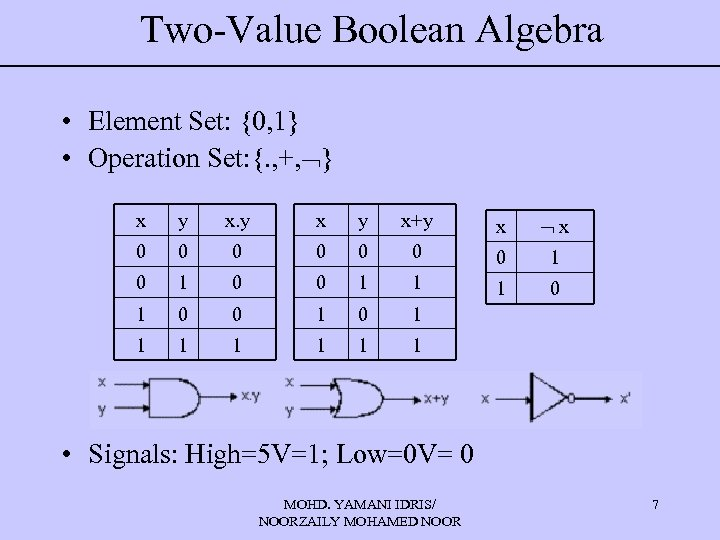Two-Value Boolean Algebra • Element Set: {0, 1} • Operation Set: {. , +, } x y x+y x x 0 0 0 0 1 1 1 0 0 1 1 1 1 • Signals: High=5 V=1; Low=0 V= 0 MOHD. YAMANI IDRIS/ NOORZAILY MOHAMED NOOR 7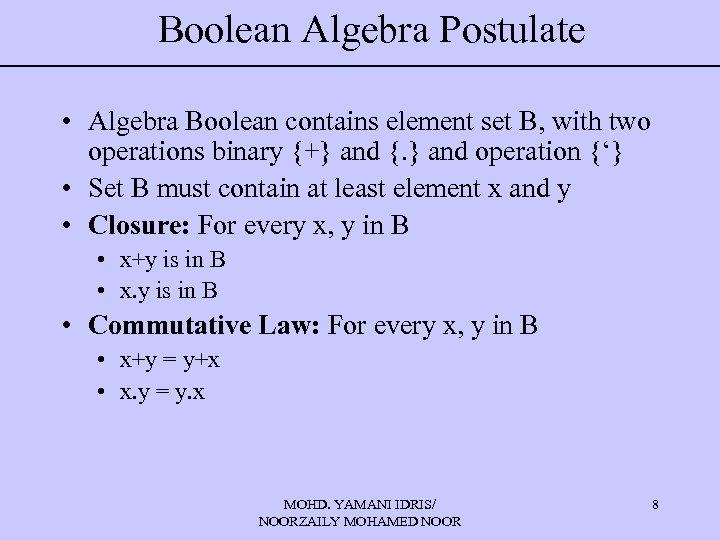Boolean Algebra Postulate • Algebra Boolean contains element set B, with two operations binary {+} and {. } and operation {‘} • Set B must contain at least element x and y • Closure: For every x, y in B • x+y is in B • x. y is in B • Commutative Law: For every x, y in B • x+y = y+x • x. y = y. x MOHD. YAMANI IDRIS/ NOORZAILY MOHAMED NOOR 8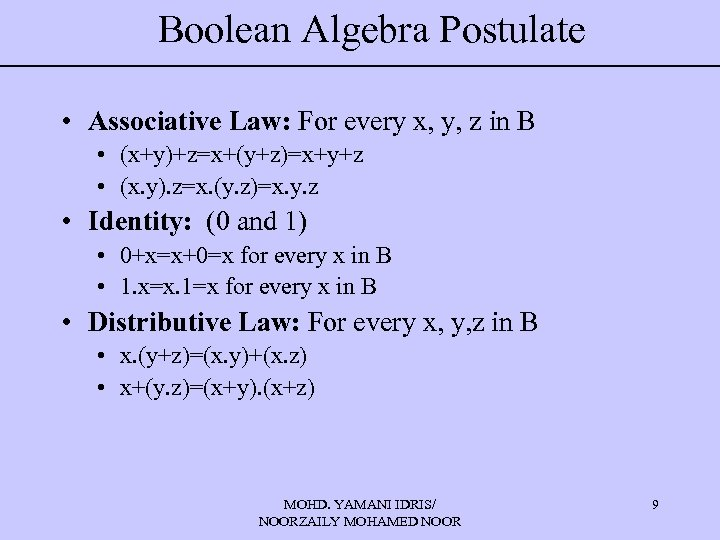Boolean Algebra Postulate • Associative Law: For every x, y, z in B • (x+y)+z=x+(y+z)=x+y+z • (x. y). z=x. (y. z)=x. y. z • Identity: (0 and 1) • 0+x=x+0=x for every x in B • 1. x=x. 1=x for every x in B • Distributive Law: For every x, y, z in B • x. (y+z)=(x. y)+(x. z) • x+(y. z)=(x+y). (x+z) MOHD. YAMANI IDRIS/ NOORZAILY MOHAMED NOOR 9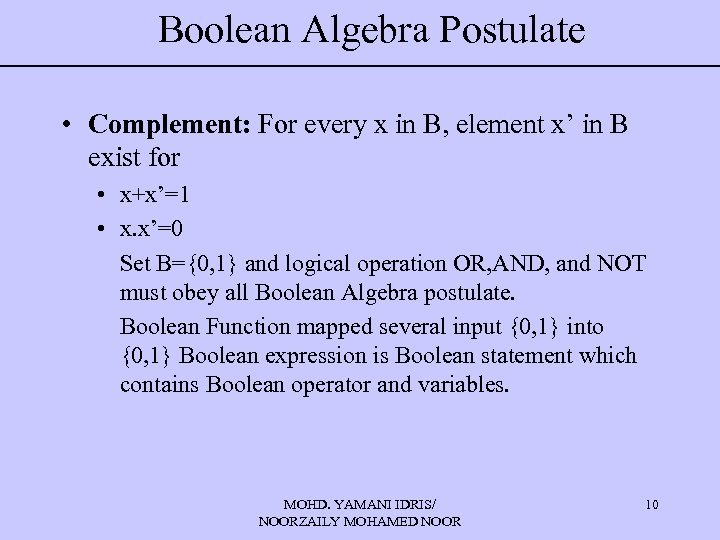Boolean Algebra Postulate • Complement: For every x in B, element x’ in B exist for • x+x’=1 • x. x’=0 Set B={0, 1} and logical operation OR, AND, and NOT must obey all Boolean Algebra postulate. Boolean Function mapped several input {0, 1} into {0, 1} Boolean expression is Boolean statement which contains Boolean operator and variables. MOHD. YAMANI IDRIS/ NOORZAILY MOHAMED NOOR 10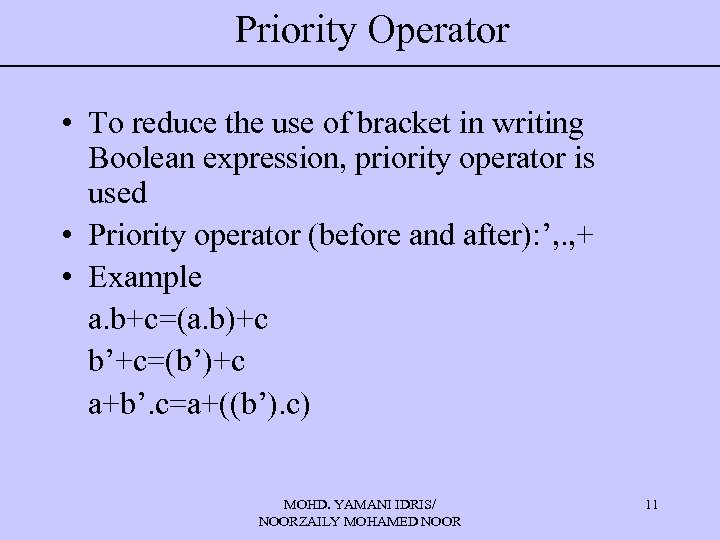Priority Operator • To reduce the use of bracket in writing Boolean expression, priority operator is used • Priority operator (before and after): ’, . , + • Example a. b+c=(a. b)+c b’+c=(b’)+c a+b’. c=a+((b’). c) MOHD. YAMANI IDRIS/ NOORZAILY MOHAMED NOOR 11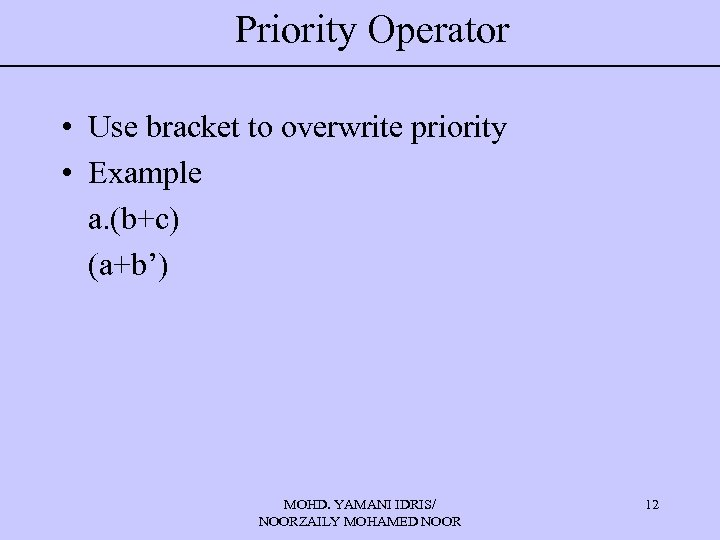Priority Operator • Use bracket to overwrite priority • Example a. (b+c) (a+b’) MOHD. YAMANI IDRIS/ NOORZAILY MOHAMED NOOR 12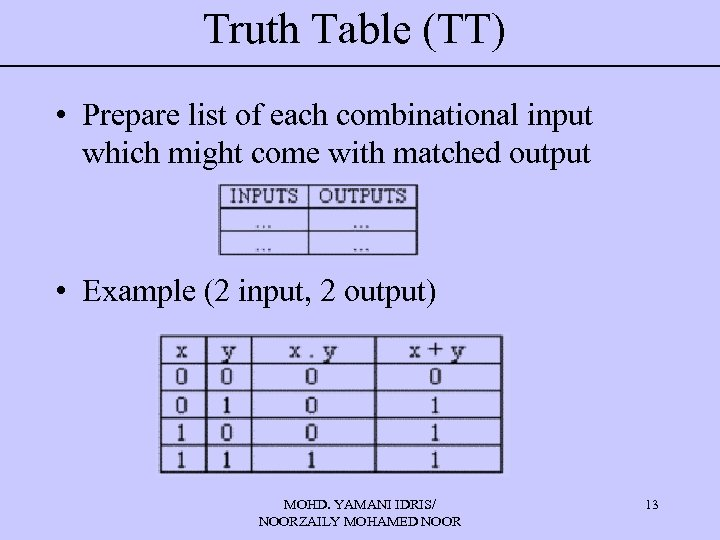Truth Table (TT) • Prepare list of each combinational input which might come with matched output • Example (2 input, 2 output) MOHD. YAMANI IDRIS/ NOORZAILY MOHAMED NOOR 13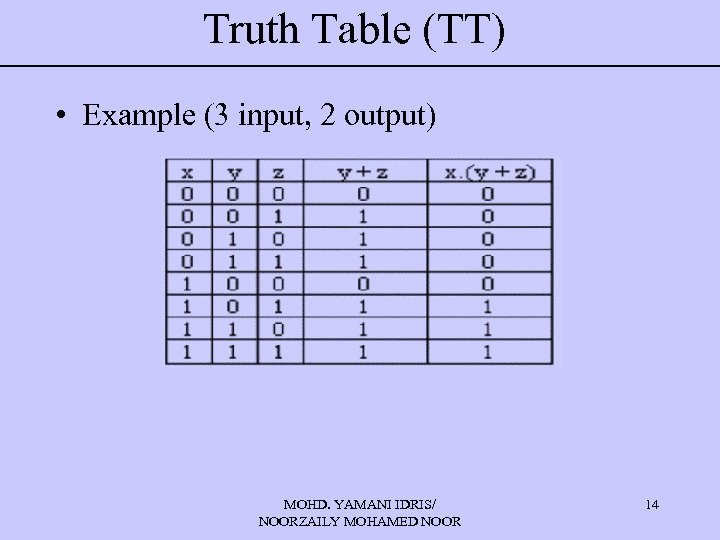Truth Table (TT) • Example (3 input, 2 output) MOHD. YAMANI IDRIS/ NOORZAILY MOHAMED NOOR 14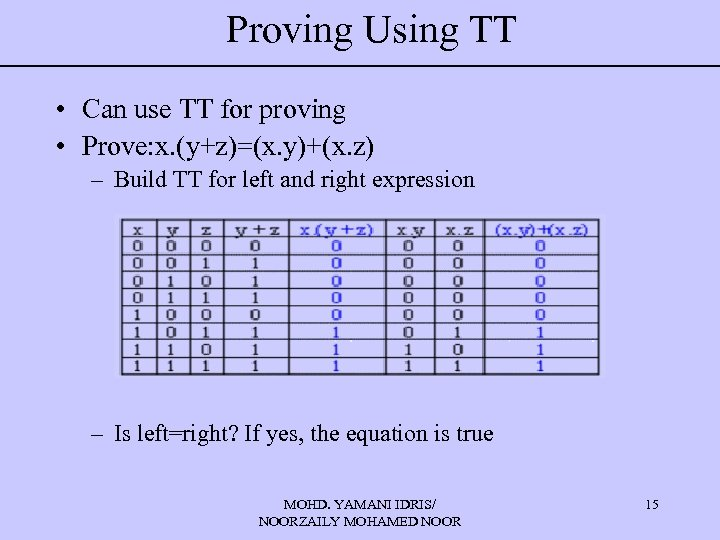Proving Using TT • Can use TT for proving • Prove: x. (y+z)=(x. y)+(x. z) – Build TT for left and right expression – Is left=right? If yes, the equation is true MOHD. YAMANI IDRIS/ NOORZAILY MOHAMED NOOR 15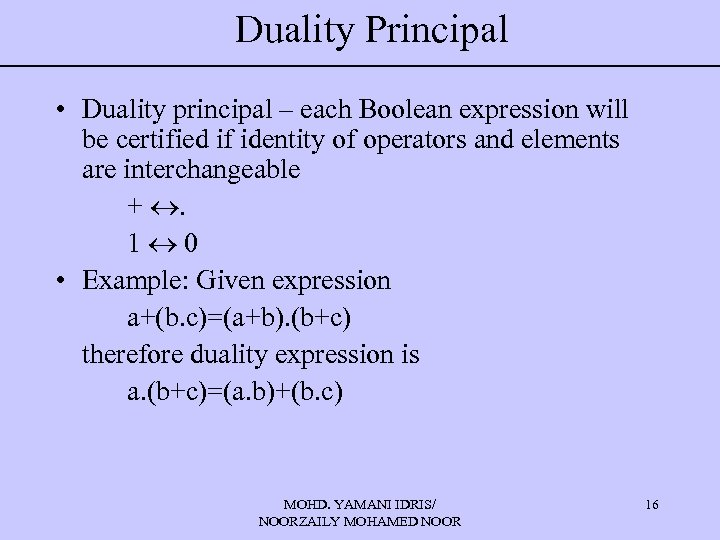Duality Principal • Duality principal – each Boolean expression will be certified if identity of operators and elements are interchangeable + . 1 0 • Example: Given expression a+(b. c)=(a+b). (b+c) therefore duality expression is a. (b+c)=(a. b)+(b. c) MOHD. YAMANI IDRIS/ NOORZAILY MOHAMED NOOR 16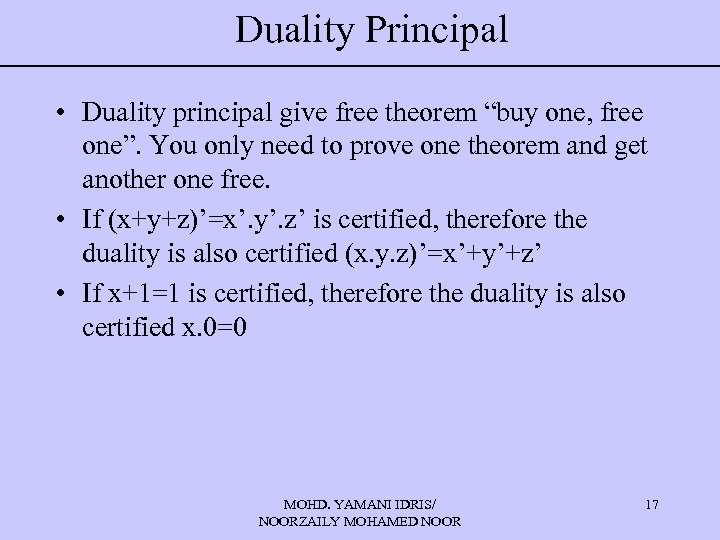Duality Principal • Duality principal give free theorem “buy one, free one”. You only need to prove one theorem and get another one free. • If (x+y+z)’=x’. y’. z’ is certified, therefore the duality is also certified (x. y. z)’=x’+y’+z’ • If x+1=1 is certified, therefore the duality is also certified x. 0=0 MOHD. YAMANI IDRIS/ NOORZAILY MOHAMED NOOR 17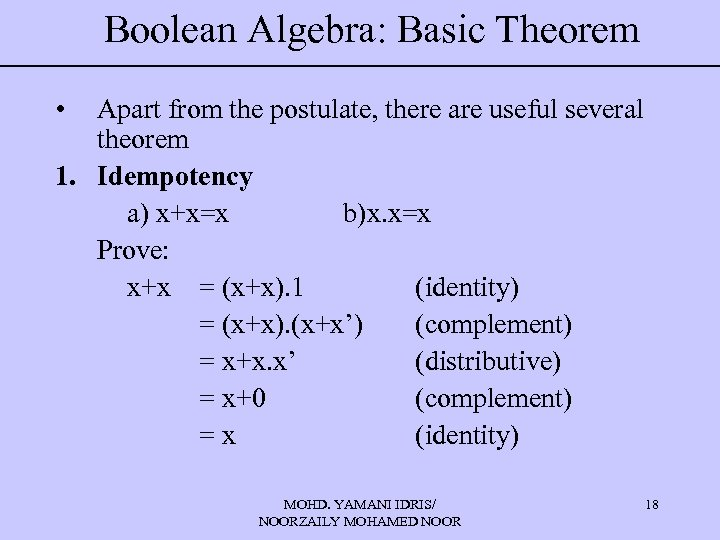Boolean Algebra: Basic Theorem • Apart from the postulate, there are useful several theorem 1. Idempotency a) x+x=x b)x. x=x Prove: x+x = (x+x). 1 (identity) = (x+x). (x+x’) (complement) = x+x. x’ (distributive) = x+0 (complement) =x (identity) MOHD. YAMANI IDRIS/ NOORZAILY MOHAMED NOOR 18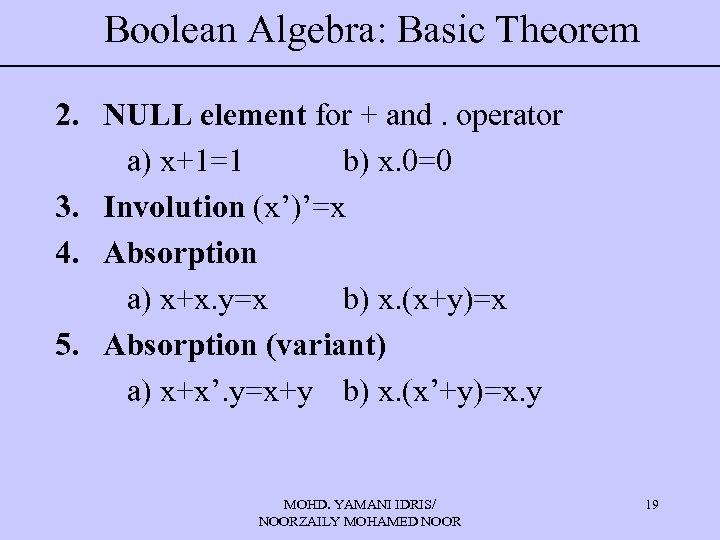Boolean Algebra: Basic Theorem 2. NULL element for + and. operator a) x+1=1 b) x. 0=0 3. Involution (x’)’=x 4. Absorption a) x+x. y=x b) x. (x+y)=x 5. Absorption (variant) a) x+x’. y=x+y b) x. (x’+y)=x. y MOHD. YAMANI IDRIS/ NOORZAILY MOHAMED NOOR 19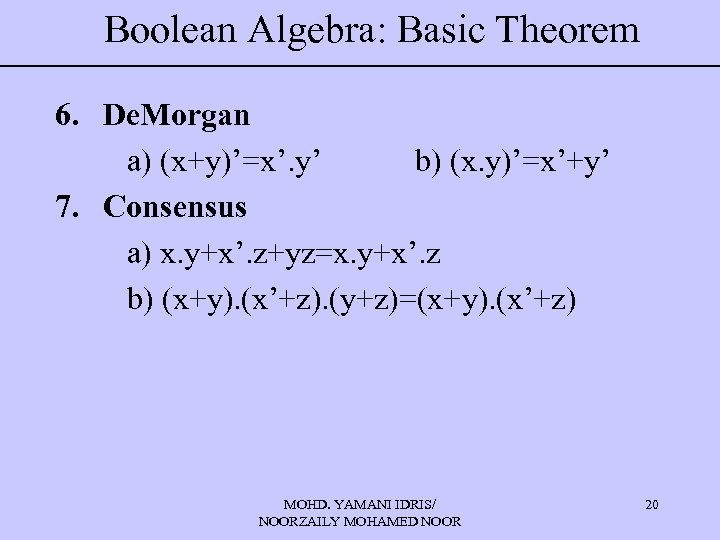Boolean Algebra: Basic Theorem 6. De. Morgan a) (x+y)’=x’. y’ b) (x. y)’=x’+y’ 7. Consensus a) x. y+x’. z+yz=x. y+x’. z b) (x+y). (x’+z). (y+z)=(x+y). (x’+z) MOHD. YAMANI IDRIS/ NOORZAILY MOHAMED NOOR 20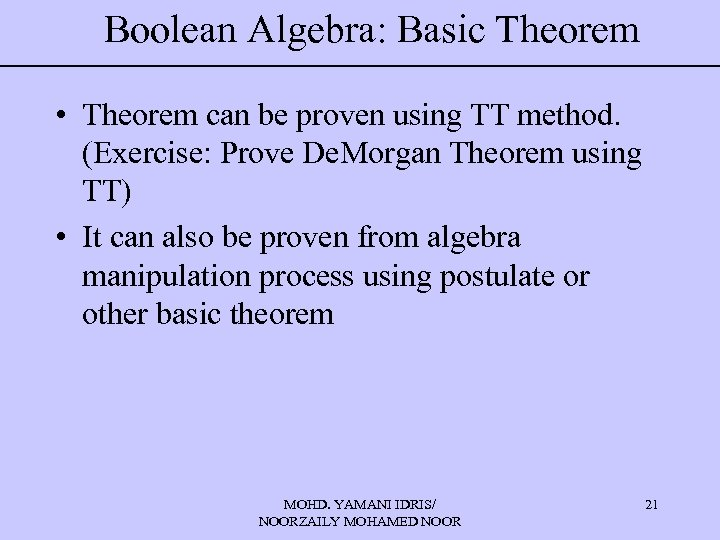Boolean Algebra: Basic Theorem • Theorem can be proven using TT method. (Exercise: Prove De. Morgan Theorem using TT) • It can also be proven from algebra manipulation process using postulate or other basic theorem MOHD. YAMANI IDRIS/ NOORZAILY MOHAMED NOOR 21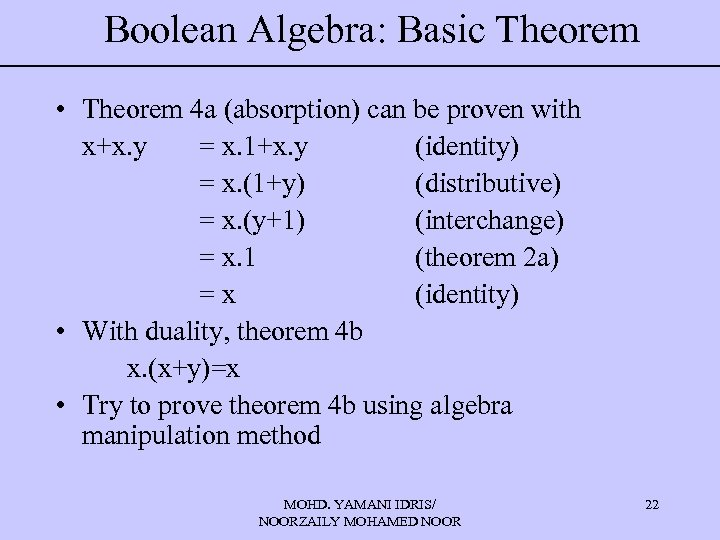Boolean Algebra: Basic Theorem • Theorem 4 a (absorption) can be proven with x+x. y = x. 1+x. y (identity) = x. (1+y) (distributive) = x. (y+1) (interchange) = x. 1 (theorem 2 a) =x (identity) • With duality, theorem 4 b x. (x+y)=x • Try to prove theorem 4 b using algebra manipulation method MOHD. YAMANI IDRIS/ NOORZAILY MOHAMED NOOR 22